# Inlets & outlets¶

Inlets are engines which generate new particles during the simulation; their counterpart are outlets (BoxOutlet) deleting particles during the simulation.

Inlets are split into several orthogonal components:

1. ParticleGenerator creating a new particle, given some input criteria, such as the PSD;
2. Inlet, which positions the new particle, given some spatial requirements (random, given sequence and such);
3. ParticleShooter is used with random inlets and assigns an intial velocity to the particle; this component is optional, and actually only rarely used.

## Generators¶

### PSD¶

Particle size distribution (PSD) is an important characteristics of granular materials – cumulative distribution function (CDF) statistically describing distribution of grain sizes. In Woo, PSD is given as points psdPts of a piecewise-linear function. The $$x$$-values should be increasing and $$y$$-values should be non-decreasing (increasing or equal). The $$y$$-value used for the last point is always taken as 100%, and all other $$y$$-values are re-scaled accordingly.

For example, let’s have the following PSD:Let’s play with the generator now:

Woo: gen=PsdSphereGenerator(psdPts=[(.2,0),(.3,.2),(.4,.9),(.5,1.)])

# generators can be called using (material)
# let's do that in the loop, discaring the result (new particles)
# the generator remembers the diameter internally
Woo: m=FrictMat()  # create any material, does not matter now

# run the generator 10000 times
Woo: for i in range(0,10000): gen(m)

# plot normalized PSD
Woo: pylab.figure(3); \
...: pylab.plot(*gen.inputPsd(),label= 'in'); \
...: pylab.plot(*gen.     psd(),label='out'); \
...: pylab.legend(loc='best');
...:

# let's define this function for the following
Woo: def genPsdPlot(g,**kw):
...:   pylab.figure(max(pylab.get_fignums())+1)  # new figure
...:   pylab.plot(*g.inputPsd(**kw),label= 'in')
...:   pylab.plot(*g.     psd(**kw),label='out')
...:   pylab.legend(loc='best')
...:

# plot PSD scaled by mass which was generated
Woo: genPsdPlot(gen,normalize=False)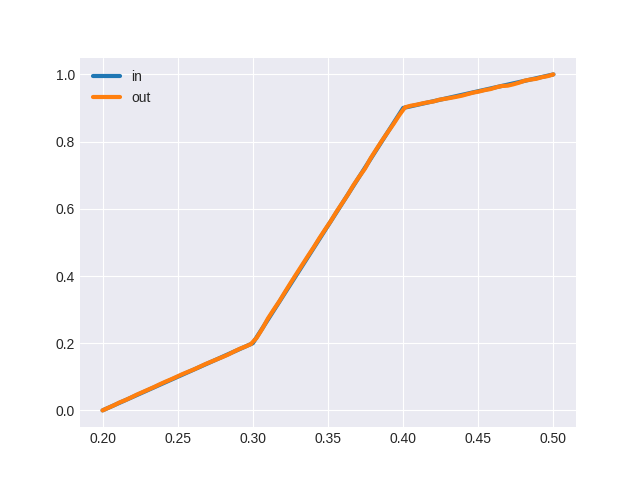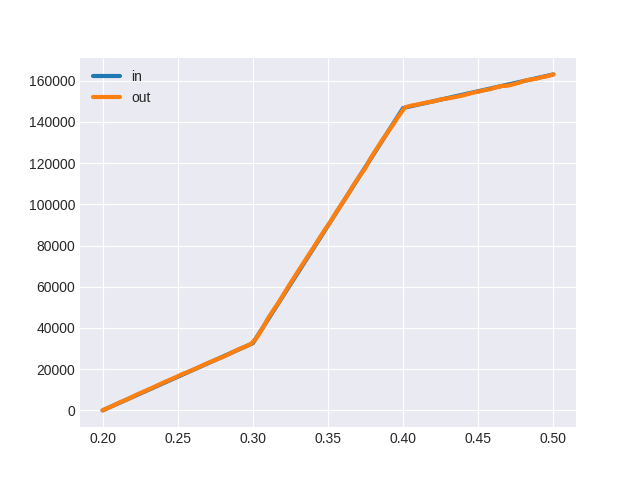The size distribution can be shown with cumulative=False:

Woo: genPsdPlot(gen,cumulative=False)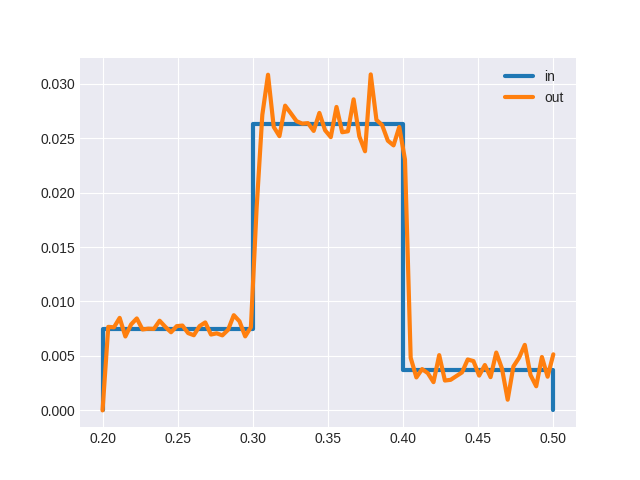#### Discrete PSD¶

The PSD is by default piecewise-linear; piecewise-constant PSD is supported by setting the discrete; discrete values of diameters will be generated. For example:

# create the discrete generator
Woo: genDis=PsdSphereGenerator(psdPts=[(.3,.2),(.4,.9),(.5,1.)],discrete=True)

# generate 10000 particles randomly
Woo: for i in range(0,10000): genDis(m)

# plot the PSD
Woo: genPsdPlot(genDis)

Woo: genPsdPlot(genDis,cumulative=False)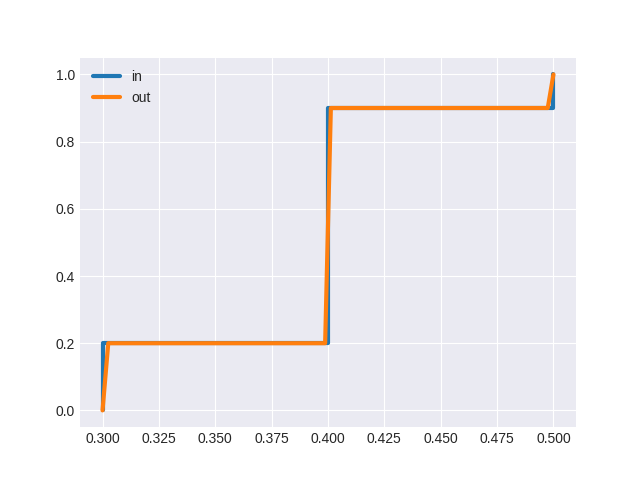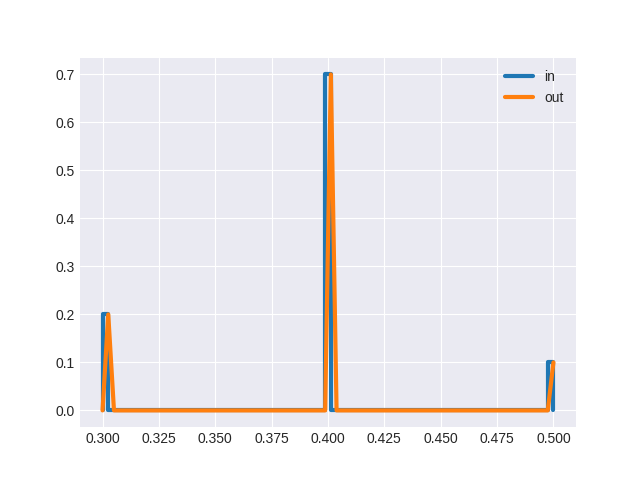#### Count-based PSD¶

In some domains, the $$y$$-axis has the meaning of cumulative count of particles rather than their mass; this is supported when mass is False; the input PSD is always plotted using the value of mass (mass-based or count-based). The output PSD can be plotted either way using the mass keyword; note that the count-based PSD is different (even if normalized) as small particles have smaller mass (proportionally to the cube of the size) than bigger ones:

# create the discrete generator
Woo: genNum=PsdSphereGenerator(psdPts=[(.3,0),(.3,.2),(.4,.9),(.5,1.)],mass=False)

Woo: for i in range(0,10000): genNum(m)

Woo: pylab.figure(max(pylab.get_fignums())+1); \
....: pylab.plot(*genNum.inputPsd(),label= 'in (count)'); \
....: pylab.plot(*genNum.psd(),label='out (mass)'); \
....: pylab.plot(*genNum.psd(mass=False),label='out (count)'); \
....: pylab.legend(loc='best');
....: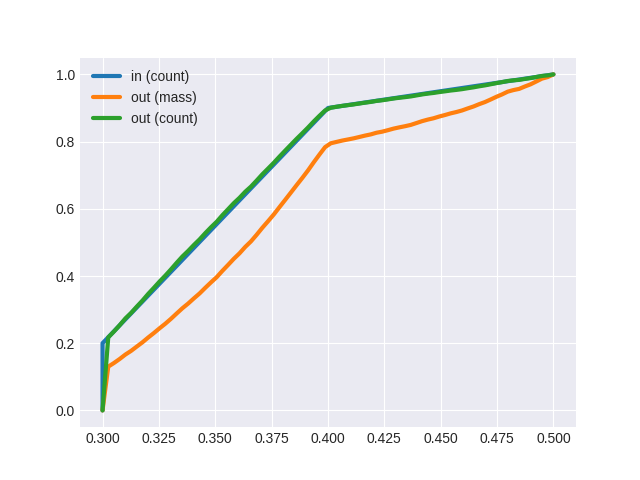#### Non-spherical particles¶

Non-spherical particles can also be generated using PSD, by using their equivalent spherical diameter (woo.dem.Shape.equivRadius gives equivalent spherical radius), i.e. diameter of sphere with the same volume. Examples of those generators are PsdEllipsoidGenerator, PsdCapsuleGenerator and PsdClumpGenerator. The clump generator can use different probabilties for different clump shapes and scale them to satisfy required PSD – see its documentation for details.

### Non-PSD¶

There are some generators which are not based on PSD; one of them is woo.dem.PharmaCapsuleGenerator which generates instances of pharmaceutical capsules of identical geometry; this generator was used in the bottle simulation shown in the previous section:

## Inlets¶

Newly created particles must be placed in the simulation space, without colliding with existing particles; this is the task of Inlet. The mass rate of particles generated can be always obtained by querying currRate.

### Random¶

Random inlets position particles in a given part of space (box with BoxInlet, 2d box with BoxInlet2d, cylinder with CylinderInlet); various parameters can govern the generation process:

1. massRate which limits how many mass per second may be generated; if set to zero, generate as many particles as “possible” in each step. “Possible” means that there will be in total maxAttempts attempts to place a particle in one step; one particle will be tried attemptPar times.
2. maxMass or maxNum limit total mass or the number of particles generated altogether. Once this limit is reached, the doneHook is run and the engine is made dead.

The bottle in the example above has CylinderInlet above the neck:

from woo.core import *
from woo.dem import *
import woo, math
from minieigen import *
# use the same material for both capsules and boundaries, for simplicity
capsMat=wallMat=woo.dem.FrictMat(ktDivKn=.2,tanPhi=.2,density=1000,young=1e7)
# create the scene object, set the master scene
S=woo.master.scene=Scene(fields=[DemField(gravity=(0,0,-10))])
# add the bottom plane (wall)
# create bottle mesh from the STL
bottle=woo.utils.importSTL('pill-bottle.coarse2.stl',mat=wallMat,scale=0.001,shift=(.056,.027,-0.01),ori=Quaternion((1,0,0),math.pi/2.),color=-.25)
# create node which will serve as "handle" to move the bottle
S.lab.botNode=Node(pos=(0,0,.04),dem=ClumpData(blocked='xyzXYZ'))
# center is the centroid normally, but the mesh has no mass, thus reference point must be given

S.dtSafety=.5

import woo.gl
woo.gl.Renderer.engines=False
woo.gl.Renderer.allowFast=False

# particle factory, with many parameters
# when the factory finishes, it will call the pillsDone function, defined below

# add factory (and optional Paraview export) to engines
S.engines=DemField.minimalEngines(damping=.3)+[
factory,
## comment out to enable export for Paraview:
VtkExport(out='/tmp/bottle.',ascii=True,stepPeriod=500)
]
# save the scene
S.saveTmp()

def pillsDone(S):
# once the bottle is full, wait for another 0.2s
#then start moving the bottle by interpolating prescribed positions and orientations
S.lab.botNode.dem.impose=InterpolatedMotion(t0=S.time+0.2,poss=[S.lab.botNode.pos,(0,.05,.08),(0,.05,.09),(0,.04,.13)],oris=[Quaternion.Identity,((1,0,0),.666*math.pi),((1,0,0),.85*math.pi),((1,0,0),.9*math.pi)],times=[0,.3,.5,1.6])


#### Spatial bias¶

Sometimes, it is useful to distribute particles in the inlet volume non-uniformly, such as by requiring larger radii to tend to one end; this allows to produce graded or layered packings, e.g. for use in geomechanics. This functionality is provided by subclasses of SpatialBias, in particular by PsdAxialBias.

All random inlets (see below) pick point uniformly from unit cube initially, and then map it on the area they occupy (box for BoxInlet, arc for ArcInlet) by transforming coordinates (cartesian or cylindrical, respectively). PsdAxialBias will change one of the coordinates (chosen by axis) depending on diameter of the particle being generated. The mapping function is PSD-like (monotonically increasing, providing the desired unit coordinate for each diameter), and indeed the PSD can be re-used between the generator and the bias.

• fuzz can be given to introduce some random perturbation to the coordinate computed.
• the PSD-like function can be inverted to be monotonically decreasing rather than increasing;
• segments of the PSD-like sequence can be reordered to allow arbitrary arrangement of layers.
• discrete PSD’s are supported, and each of the few diameters generated will occupy space between the last fraction and its own.

The script below gives the following, showing some of the possibilities: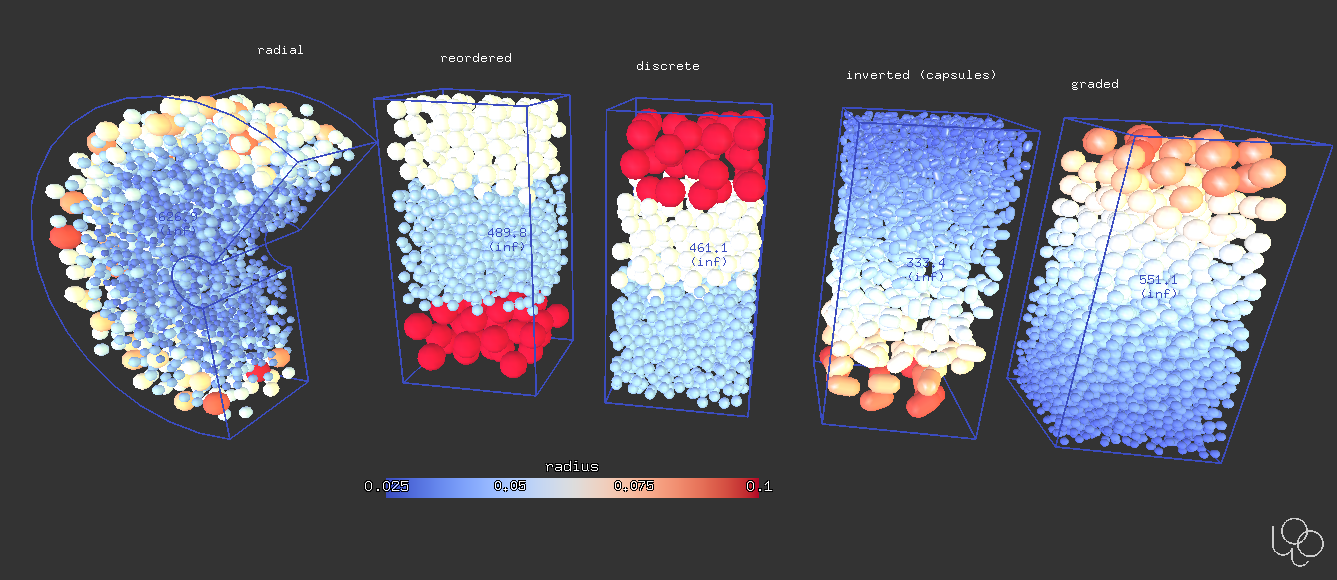Fig. 7 Various possibilities for the inlet bias functionality.

import woo.dem, woo.core, woo.log, math
from minieigen import *
S=woo.master.scene=woo.core.Scene(fields=[woo.dem.DemField()])

psd=[(.05,0),(.08,.4),(.12,.7),(.2,1)]

# attributes common to all inlets
inletKw=dict(maxMass=-1,maxNum=-1,massRate=0,maxAttempts=5000,materials=[woo.dem.FrictMat(density=1000,young=1e4,tanPhi=.9)])

S.engines=[
woo.dem.InsertionSortCollider([woo.dem.Bo1_Sphere_Aabb()]),
# regular bias
woo.dem.BoxInlet(
box=((0,0,0),(1,1,2)),
generator=woo.dem.PsdSphereGenerator(psdPts=psd,discrete=False,mass=True),
spatialBias=woo.dem.PsdAxialBias(psdPts=psd,axis=2,fuzz=.1,discrete=False),
**inletKw
),
# inverted bias, with capsules
woo.dem.BoxInlet(
box=((1.5,0,0),(2.5,1,2)),
spatialBias=woo.dem.PsdAxialBias(psdPts=psd,axis=2,fuzz=.1,invert=True),
**inletKw
),
# discrete PSD
woo.dem.BoxInlet(
box=((3,0,0),(4,1,2)),
generator=woo.dem.PsdSphereGenerator(psdPts=psd,discrete=True),
spatialBias=woo.dem.PsdAxialBias(psdPts=psd,axis=2,fuzz=.1,discrete=True),
**inletKw
),
# reordered discrete PSD
woo.dem.BoxInlet(
box=((4.5,0,0),(5.5,1,2)),
generator=woo.dem.PsdSphereGenerator(psdPts=psd,discrete=True),
spatialBias=woo.dem.PsdAxialBias(psdPts=psd,axis=2,discrete=True,reorder=[2,0,1,3]),
**inletKw
),
# radial bias in arc inlet
woo.dem.ArcInlet(
node=woo.core.Node(pos=(7,1,.65),ori=Quaternion((1,0,0),math.pi/2)),cylBox=((.2,-math.pi/2,0),(1.3,.75*math.pi,1)),
generator=woo.dem.PsdSphereGenerator(psdPts=psd),
spatialBias=woo.dem.PsdAxialBias(psdPts=psd,axis=0,fuzz=.7),
**inletKw
),
]

# actually generate the particles
S.one()

woo.gl.Renderer.iniViewDir=(0,-1,0)

# abuse DEM nodes to stick labels on the top
S.dem.nodesAppend(woo.core.Node((1+i*1.5,0,2.2),dem=woo.dem.DemData(),rep=woo.gl.LabelGlRep(text=t)))


### Dense¶

There is a special inlet ConveyorInlet which can continuously produce stream of particles from pre-generated (compact, dense) arrangement which is periodic in one direction; as the name suggests, this is especially useful for simulating conveyors. The compaction process is itself dynamic simulation, which generates particles randomly in space at first, and lets them settle down in gravity; all this happens behind the scenes in the woo.pack.makeBandFeedPack function.

Note

Since the initial compaction is a relatively expensive (time-demanding) process, use the memoizeDir argument so that when the function is called with the same argument, it returns the result immediately.

import woo, woo.pack, woo.utils
from woo.core import *
from woo.dem import *
from minieigen import *

# some material that we will use
mat=woo.utils.defaultMaterial()

# create the compact packing which will be used by the factory
sp=woo.pack.makeBandFeedPack(
# dim: x-size, y-size, z-size
# memoizeDir: cache result so that same input parameters return the result immediately
dim=(.3,.7,.3),mat=mat,gravity=(0,0,-9.81),memoizeDir='/tmp',
# generator which determines which particles will be there
)

# create new scene
S=woo.master.scene=woo.core.Scene(fields=[DemField(gravity=(0,0,-10))])
# inially only the plane is present

S.engines=DemField.minimalEngines(damping=.2)+[
# this is the factory engine
ConveyorInlet(
stepPeriod=100,     # run every 100 steps
shapePack=sp,       # this is the packing which will be used
vel=1.,             # linear velocity of particles
maxMass=150,        # finish once this mass was generated
doneHook='S.stop()',# run this once we finish
material=mat,       # material for new particles
# node which determines local coordinate system (particles move along +x initially)
node=Node(pos=(0,0,.2),ori=Quaternion((0,-1,0),.5)),
)
]

S.saveTmp()
#S.run()
#S.wait()



This is another movie (created in Paraview) showing the ConveyorInlet with spherical particles:

## Shooters¶

When random inlets generate particles, they have zero intial velocity; this can be changed by assigning a ParticleShooter to shooter, as in this example:

import woo, woo.pack, woo.utils; from woo.core import *; from woo.dem import *; from minieigen import *

S=woo.master.scene=woo.core.Scene(fields=[DemField(gravity=(0,0,-10))])

S.engines=DemField.minimalEngines(damping=.2)+[
BoxInlet(
stepPeriod=100,                           # run every 100 steps
box=((0,0,0),(1,1,1)),                    # unit box for new particles
massRate=2.,                              # limit rate of new particles
materials=[woo.utils.defaultMaterial()],  # some material here
# spheres with diameters distributed uniformly in dRange
generator=MinMaxSphereGenerator(dRange=(.02,.05)),
# assign initial velocity in the given direction, with the magnitude in vRange
shooter=AlignedMinMaxShooter(vRange=(1.,1.5),dir=(0,.7,.5)),
label='feed'
)
]
S.saveTmp()


## Outlets¶

The inverse task is to delete particles from simulation while it is running; this is done using the woo.dem.BoxOutlet engine. This engine is able to report PSD just like inlets. Comparing PSDs from inlet and from the outlet can be useful for segregation analysis.

from past.builtins import execfile
# use the setup from the other file
execfile('factory-shooter.py')
# replace the generator
# increase its mass rate
S.lab.feed.massRate=10
# necessary for capsules... ?
S.dtSafety=0.4
S.engines=S.engines+[
BoxOutlet(
box=((0,0,0),(1,1.1,1)),
stepPeriod=100,
save=True,      # keep track of diameters/masses of deleted particles, for PSD
inside=False,   # delete particles outside the box, not inside
label='out'
)
]
S.saveTmp()

if 1:
S.run(30000)
S.wait()

# plots PSDs
import pylab
pylab.plot(*S.lab.feed.generator.psd(),label='feed',lw=2)
pylab.plot(*S.lab.out.psd(),label='out',lw=2)
pylab.legend(loc='best')
# pylab.show()
pylab.savefig('fig/factory-deleter_psds.svg')
pylab.savefig('fig/factory-deleter_psds.pdf')Fig. 8 Comparison of PSDs of the inlet and the outlet.

Tip

Ask questions or report issues at github.